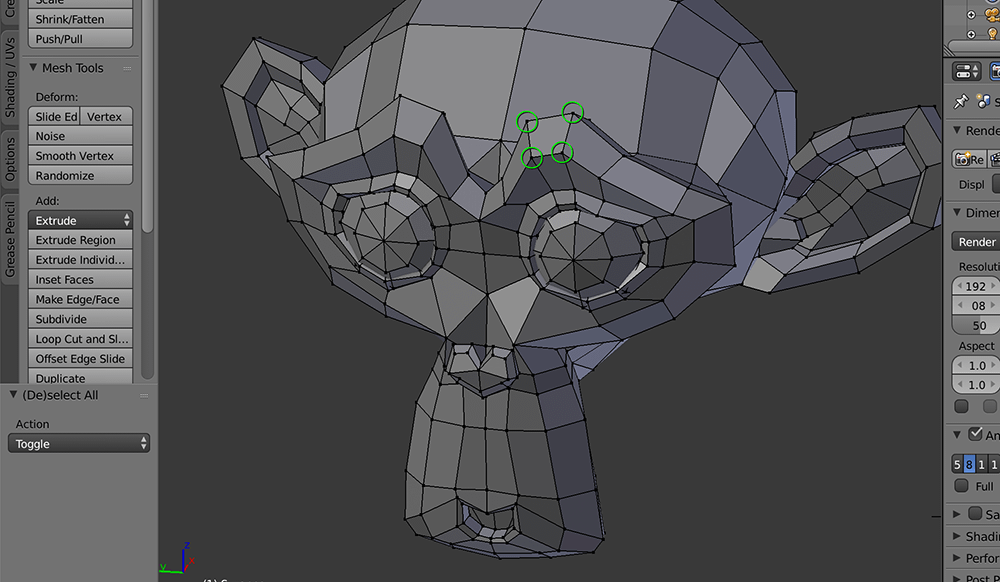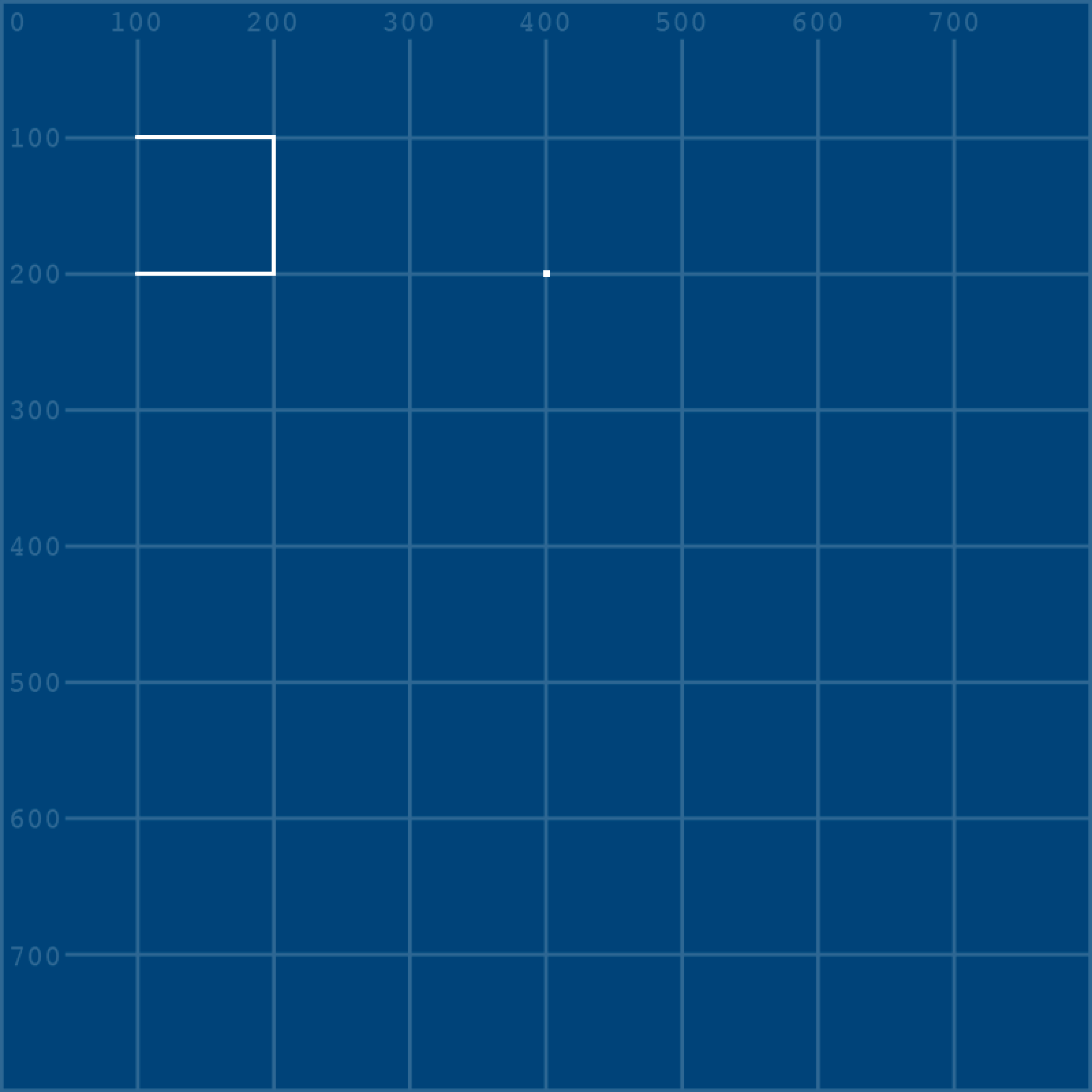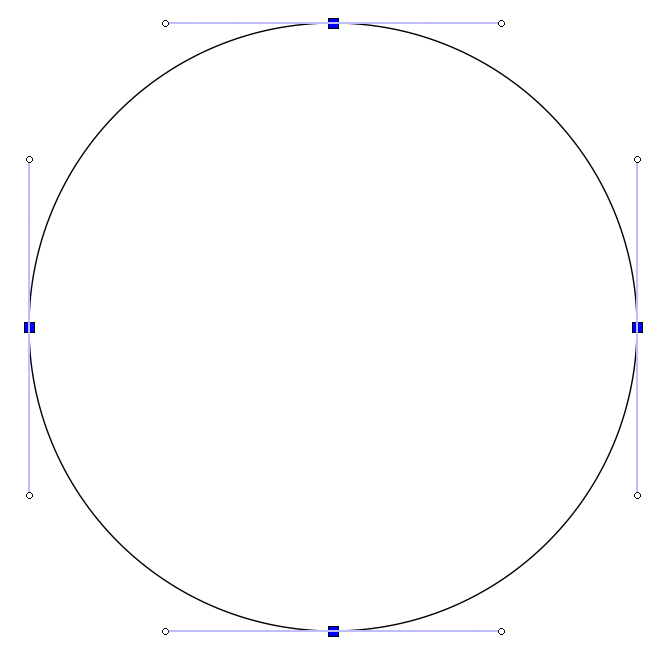tabreturn.github.io

# Processing.py in Ten Lessons – 2.2: Vertices

2018-06-20

## Vertices

You can think of vertices as the dots in a connect-the-dots style drawing puzzle. A triangle requires three vertices; a pentagon, five; a five-pointed star (★), ten; and so forth. By connecting vertices using lines and curves, the shape possibilities become limitless. A vertex (singular) is not limited to two-dimensional space – for instance, Blender’s Suzanne (a monkey head) has around five-hundred vertices positioned in 3D space.Four – of the five-hundred, or so – vertices circled in green.

Create a new sketch and save it as “vertices”. Within the new vertices folder, add a “data” folder containing the grid.png file from your last sketch.

Now draw a square using vertices:

The `beginShape()` and `endShape()` functions should be self-explanatory. But, the shape will not automatically close unless you use `endShape(CLOSE)`. However, an active `fill()` will fill a shape however it can:

There are also various parameters one can provide the `beginShape()` function to determine how the enclosed vertices are connected, if at all:

For other `beginShape()` parameters, consult the reference.

### Bézier Vertices

The `bezierVertex()` function allows one to create curved shape lines. There’s also a `curveVertex()` for Catmull-Rom-type curves, but lesson 2 will focus on the Bézier type, as these allow for greater control and more graceful curves.

The `bezierVertex()` function takes the following arguments, expanded across multiple lines here for easier comprehension:

To get a better grip on how this function works, we’ll work toward completing the remaining shapes depicted below. The pale blue lines/circles provide a visual indication of where the handles and control-points lie (so there’s no need to recreate them).

You’ll be referencing this image repeatedly through this section. It may be useful to save a copy and open a preview of it alongside your Processing editor.

#### S-Bend

The S-bend is comprised of two vertices, both of which are attached to control points. Of course, this is a curve, so you could draw it using a `bezier()` function. However, the purpose of this section is to introduce shapes. Within a `beginShape()`, you can mix `bezierVertex()`, `curveVertex()` and `vertex()` however necessary. But, the first time a `bezierVertex()` is used, it must be prefaced with a `vertex()`. Begin a new shape and place the first (in this case, upper) vertex:As there's no second vertex with which to form a line, the isolated vertex appears as a point.

Now add the second vertex using `bezierVertex()`:

I’ll admit, it’s a bit confusing. But, with the positions of the vertices presented for you in the reference image, it’s really just a matter of writing in the correct sequence of coordinates.

#### Heart

You can think of the heart shape as two lines connecting two vertices. To begin, draw one half of the shape:

All that’s left for you to do is complete the right-half of the heart. Add a second `bezierVertex()` line and fill-in the arguments:

#### Chinese Coin

Round metal coins with square holes in the centre were first introduced in China many centuries ago. The violet-filled shape resembles such a coin, albeit with none of the relief/engraving. Its form requires that one shape be subtracted from another. Processing provides the `beginContour()` and `endContour()` functions for this purpose.

The first challenge is the outer circle. The contour functions are used within a `beginShape()` and `endShape()`, so using an `ellipse` function is not an option. However, circles can be drawn using Bézier curves:An Inkscape circle object converted to a path, indicating how Bézier curves are positioned to form a circle.

You can begin by forming a circle using a diamond shape:

With your vertices in place, you can now convert the `vertex()` functions to `bezierVertex()` functions. Remember, though: the first point must be a `vertex()`.

To save you having to workout where the control points lie, here are the missing arguments:

With the circle in place, you can go about removing a square from the middle. This is a relatively straightforward exercise, but there’s one crucial thing to be aware of: one must use reverse winding for the subtracted shape. Read through the circle code again and notice how all of the vertices are plotted in a clockwise manner; this means that the square’s vertices must be plotted counter-clockwise, i.e. opposite to the winding of the shape from which it will subtract.

Place the square’s vertices within a `beginContour()` and `endContour()` function. Of course, you cannot observe the effect unless you add a fill:

When you run the sketch, you’ll see four Bézier curves. Recreate them using `bezierVertex()`.